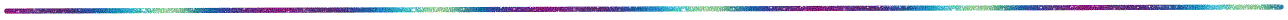# XiaLab at University of Ottawa# BIO8102L: Statitical thinking### Topics

1. Introduction to statistical thinking
2. The least-square principle in regression and beyond (and a briefing on the maximum likelihood method): EXCEL file
3. Various types of regression approaches with LAD, and LS criteria and Model II regression EXCEL file
4. Maximum likelihood criterion and its application
5. General linear models: aiming to do everything (including t-test, ANOVA, ANCOVA) by regression: I EXCEL file
6. Model selection: criteria derived from R2 and log-likelihood
7. Multiple regression with matrix approach, with EXCEL and R
8. Bayesian inference with MCMC: Lecture slides and EXCEL+R
9. Confidence interval, credible interval and high density interval: slides
10. Model II regression and Theil-Sen nonparametric regression slides
11. Bayesian regression
12. Data transformation, EXCEL file for illustrating Box-Cox power translation
13. Nonlinear regression with least-square and maximum likelihood methods: Slides and EXCEL file
14. Introduction to generalized linear models: PPT and EXCEL
15. Introduction to mixture models: PPT and EXCEL
16. Categorical data analysis: PPT slides and EXCEL file, and Iteration
17. multivariate statistics: PPT, EXCEL for manual calculation, and EXCEL with PCA, correspondence analysis, etc.